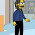## Monday, July 20, 2009

### What's wrong with this code?

```(define (& . l)
(let loop ((l l) (s ""))
(if (null? l)
s
(loop (cdr l) (string-append s (->string (car l))))))
```
The problem is that the code doesn't say what it does. Consider this version:
```(apply string-append (map ->string l))
```
We're going to take a list of items, convert them all to strings, and paste the strings together. That's pretty much what that one-liner says is going to happen. The `loop` version has a lot of other cruft in it that is the machinery to iterate down a list. We want to abstract that away.

Perhaps we don't want to use `apply` here, though. Maybe we're worried about the stack space involved. This will do the trick:
```(fold-left (lambda (s e)
(string-append s (->string e)))
""
l)
```

1.But won't the fold-left solution produce an algorithm that is O(n**2) in the number of characters in the final string?

2.The fold-left solution is identical in time and space to the named-let solution. Both are O(n^2). An O(2*N) solution is easy, but I bet you can do that both ways, too.

3.Yes, but the solution based on map should be linear, assuming that string-append with more than two arguments does the right thing.

4.Good point!

5.Actually, this big append can be linear (maybe even sub-linear). Just use ropes or finger trees for the strings (and make sure they are considered immutable).

With that, even the fold is linear, because appending two finger trees is logarithmic in the length of the smaller tree.